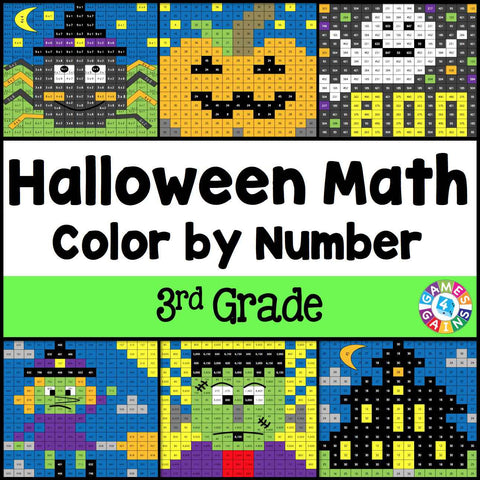## Halloween Math Color-by-Number - 3rd Grade

• \$480

Halloween Math Color-by-Number set comes with 6 Halloween math color-by-number activities for reviewing 3rd grade math skills. This Halloween math set is perfect to use in centers, in small groups, or with the whole class!

Included with this Halloween Math Color-by-Number set:
Instructions
6 color by number worksheets
6 student problem worksheets
6 teacher answer sheets

Here are the skills covered by these Halloween math color-by-number activities:

• Writing multiplication equations for arrays
• Determining the missing number while skip counting
• Solving one-step multiplication word problems (facts for 2-5, 10 only)
• Rounding numbers to the nearest ten and hundred
• Adding numbers within 1,000
• Subtracting numbers within 1,000

Want to learn more about what you'll get? Check out the preview!